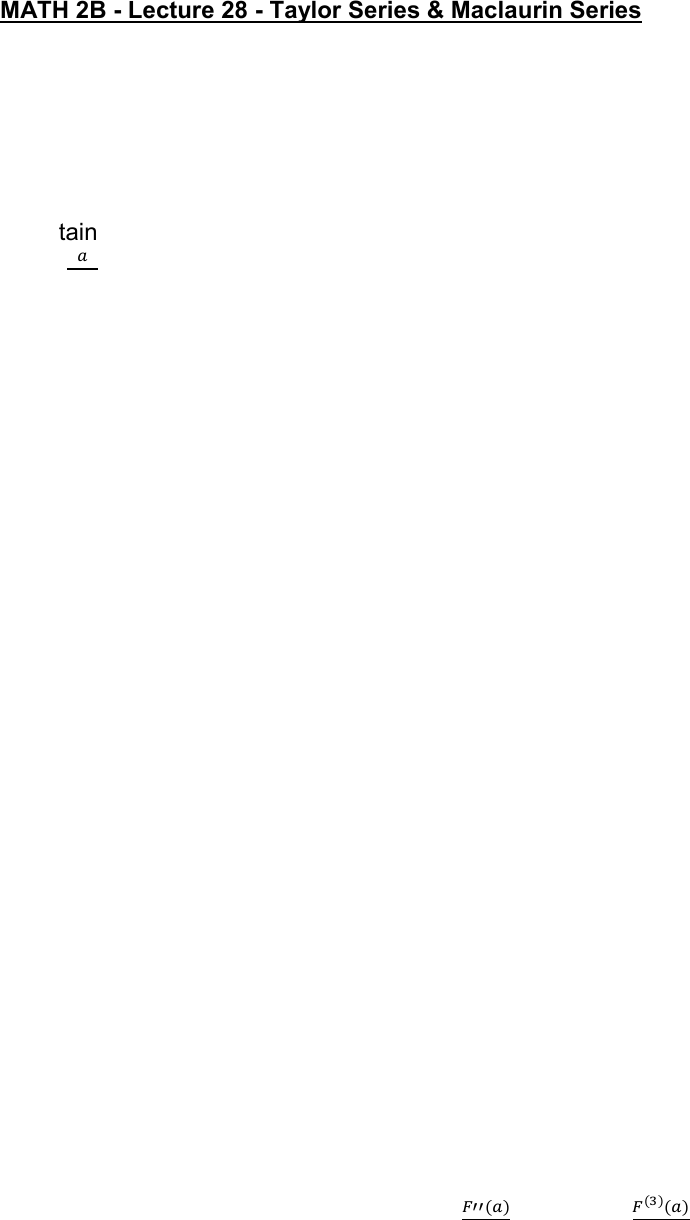Class Notes (1,100,000)
US (460,000)
UC-Irvine (10,000)
MATH (1,000)
MATH 2B (600)
Lecture 28

# MATH 2B Lecture Notes - Lecture 28: Taylor Series, Ratio TestPremium

Department
Mathematics
Course Code
MATH 2B
Professor
ERJAEE, G.
Lecture
28

This preview shows half of the first page. to view the full 3 pages of the document.03/11/2019
MATH 2B - Lecture 28 - Taylor Series & Maclaurin Series
We have learned for certain type of functions, we can use a geometric type
power series as representations.
Now we will consider a more general case, to represent a continuous F(x) by
using a general power series
Certain type “Geometric” Power Series Convergence

 
  
General Function Power Series Convergence
F(x)
    
  
Suppose we can represent some functions F(x) as power series
F(x) =
      
Now the question is how can we determine the coefficient for each term 
First, let’s write the first 5 terms of the series
      
If we plug in a for x, we notice that all the terms except the first term become zero
     
Now, we take the derivative of F(x)
      
Now we plug in a for x again, such that
  
We can further take the second derivative:
        
Plug in a for x, we obtain   
The third derivative gives us
     
Let x = a,
  
So, in summary we have:
  

